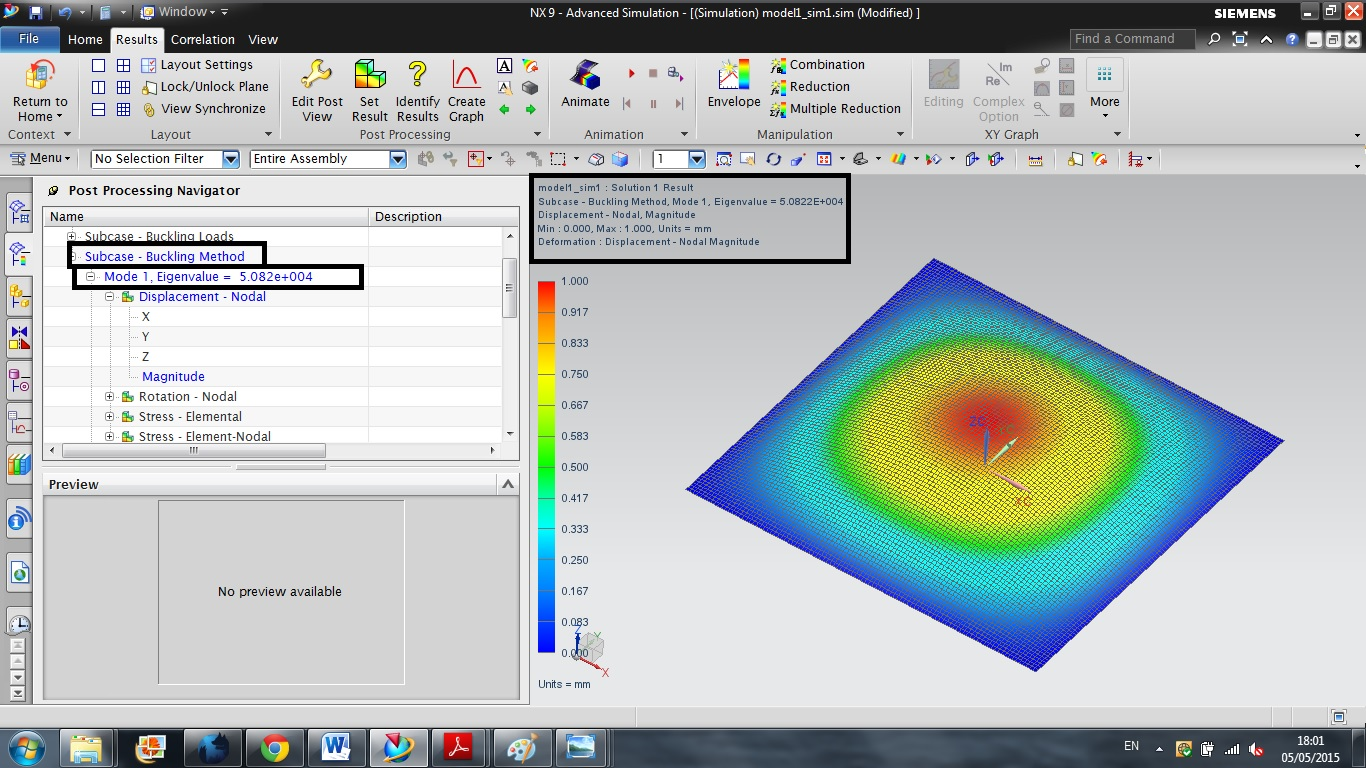# Buckling Analysis in NX software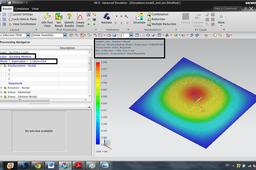This is tutorial for buckling analysis in NX 9 software. For doing this tutorial you need to have basic information of software and also you need to be familiar with buckling subject

1. ### Step 1:

In the first step you need to make geometry of the model. the thickness of a plate should be equal to : the layer thickness * the number of the layer.
In this example the 8 layer with 0.5mm thickness is considered for this sample. Therefore the thickness of the plate would be 4mm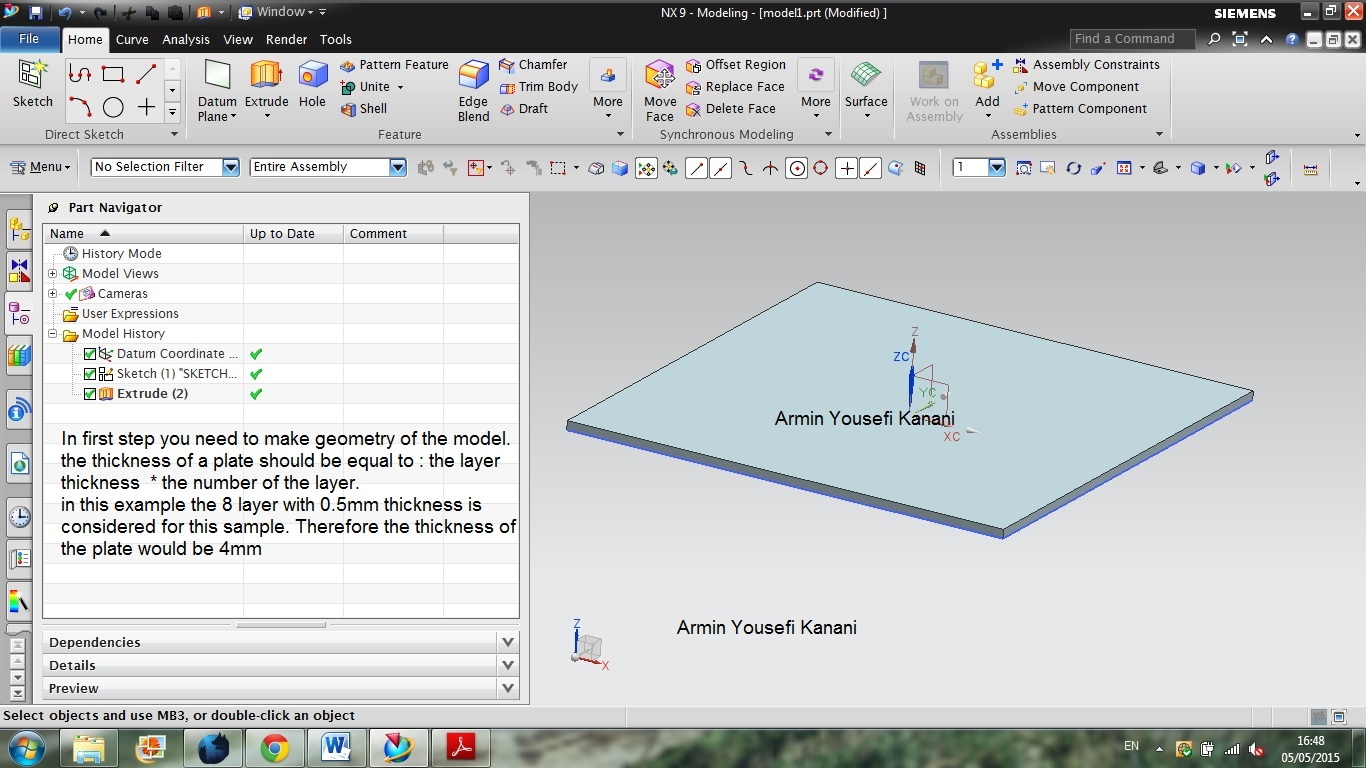2. ### Step 2:

In the second step you need to save the file and go to the advance simulation section. In this step you have to decided your analysis will be 2D or 3D. For this example 2D analysis is considered. Thus, click on the mid surface with face pair. in the solid body section choose geometry and then click on automatically create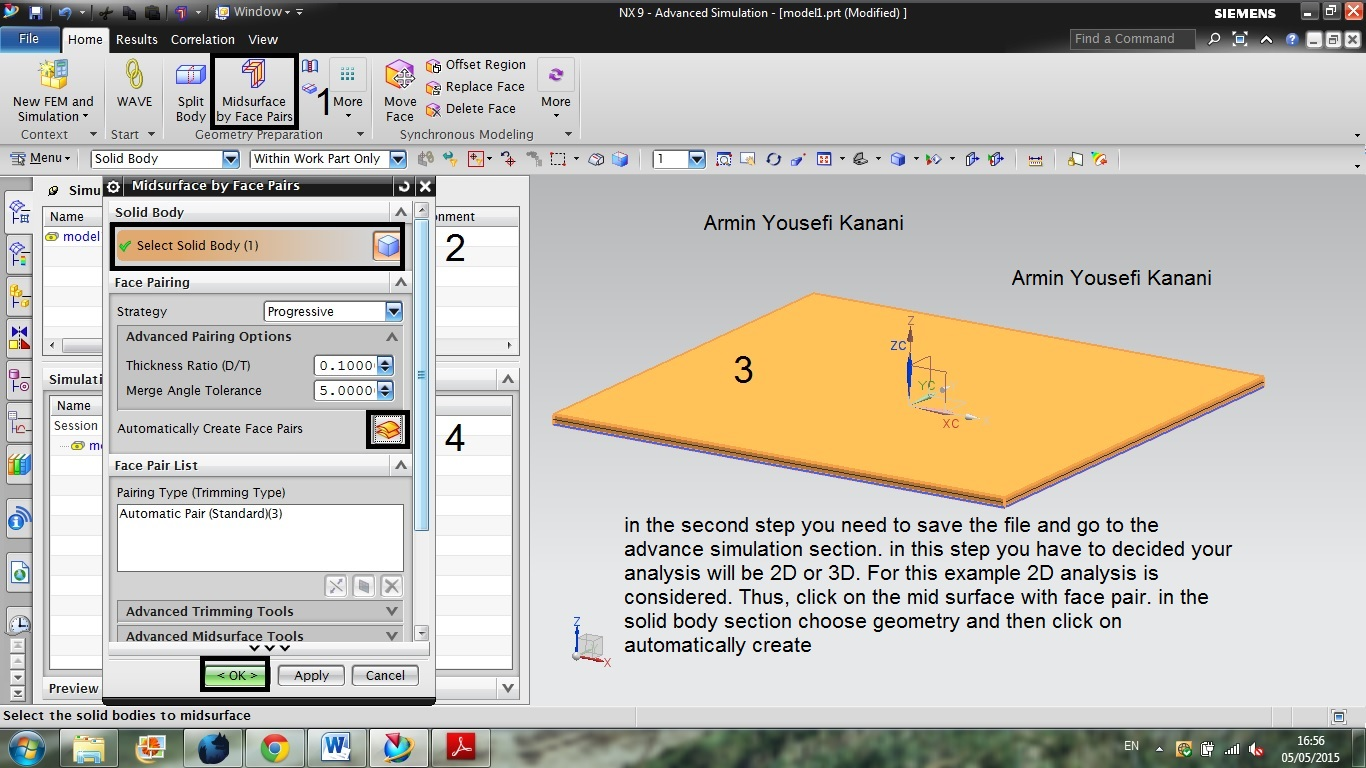3. ### Step 3:

In the step three you need to make new simulation, for this example you do not need change any option just in the solution type choose liner buckling 105.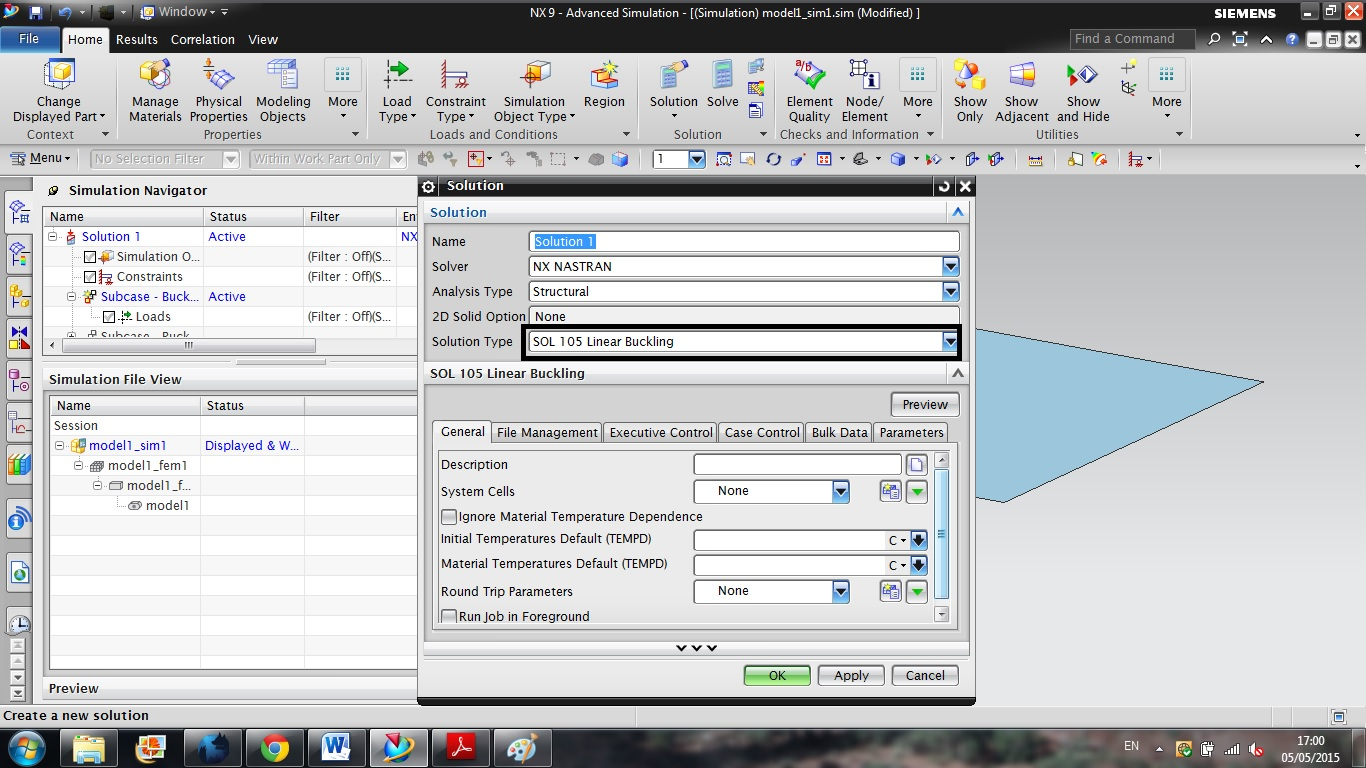4. ### Step 4:

in this section, the mesh will applied to the model. in mesh section you have to do mesh sensitivity. because by changing mesh size the eigenvalue amount will change. thus you need to find proper mesh size and mesh type. In CQUAD 4, the mesh size should not be above 5 mm but this depends on your computer to be able to process the operation.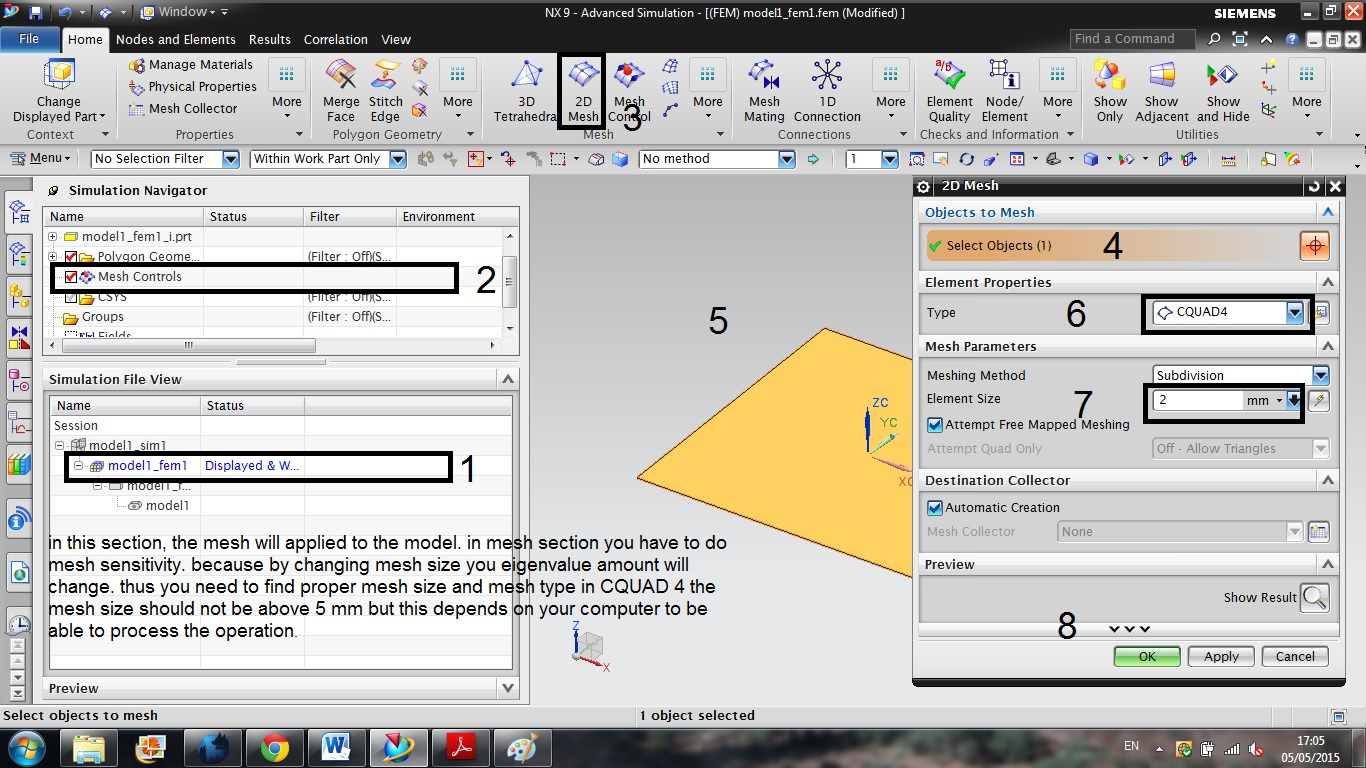5. ### Step 5:

in this section we want to enter laminate information to the software.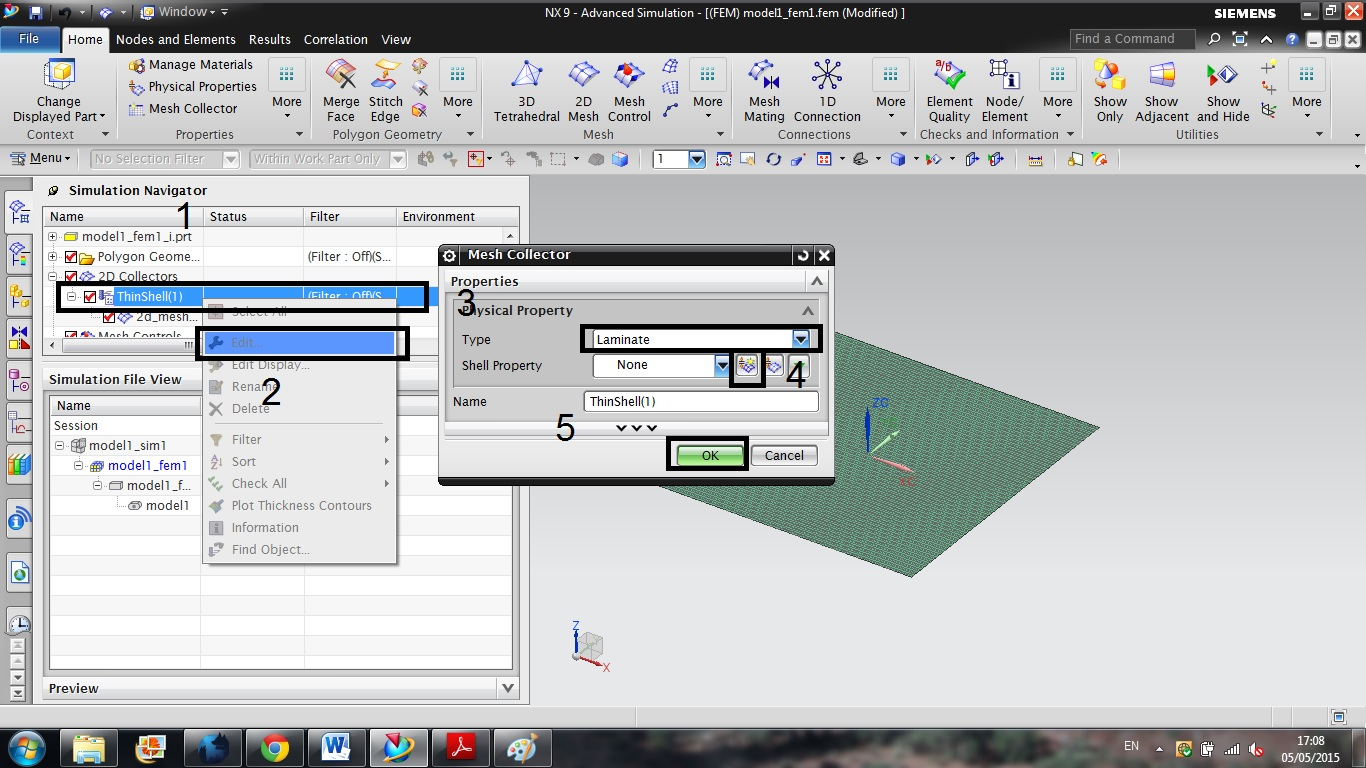6. ### Step 6:

the laminate code for this example is [(0,90)4]s, so we have 8 layers with 0.5mm thickness.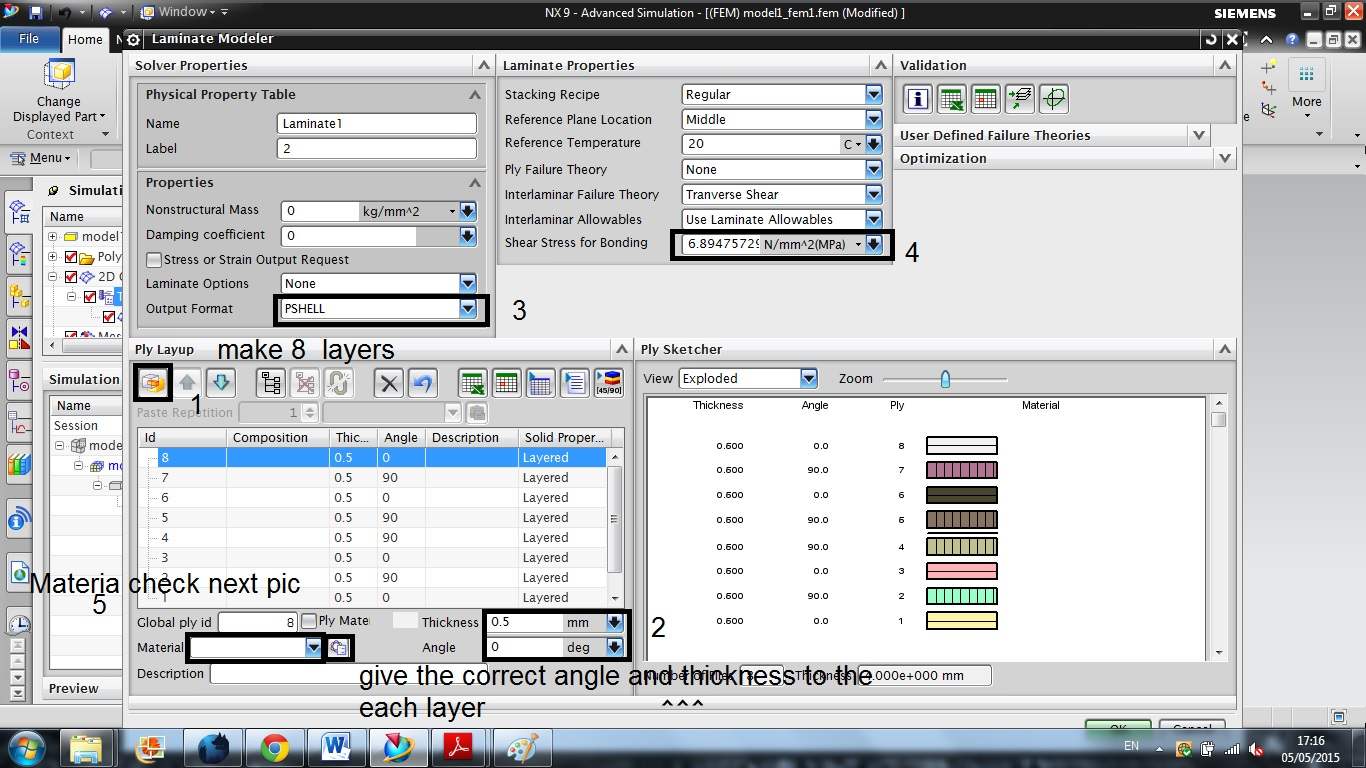7. ### Step 7:

material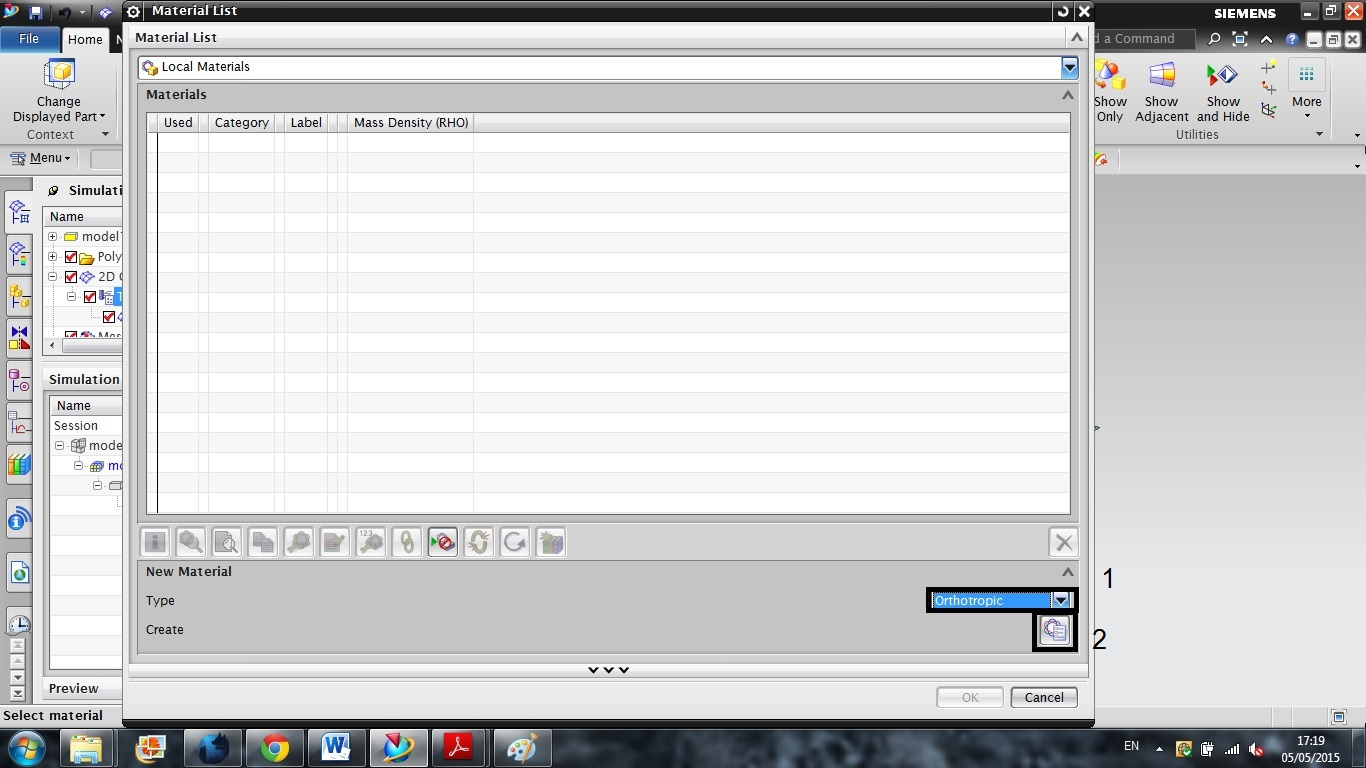8. ### Step 8:

material details
E1:172 Gpa
E2:6.89 Gpa
v12=v21=0.25

after entering information you need to give orthopedic material to each layer like previous section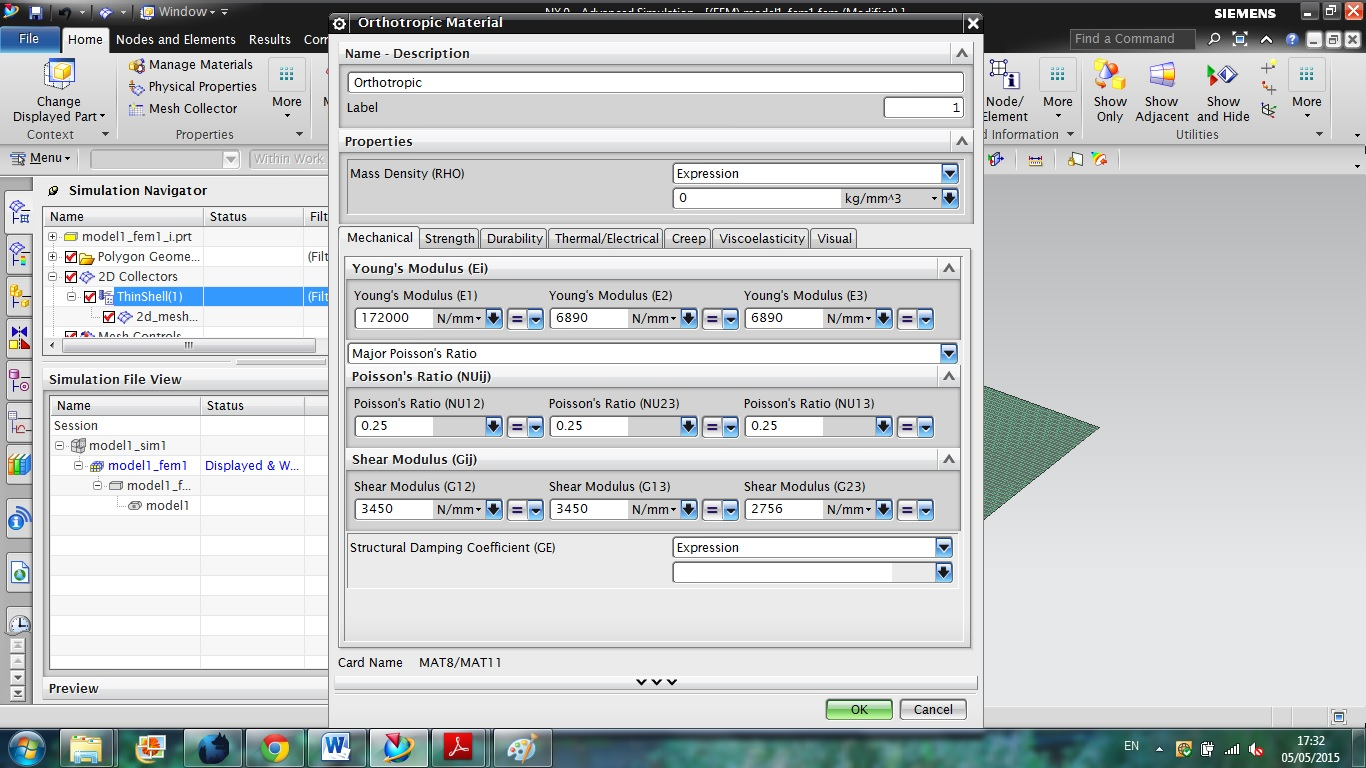9. ### Step 9:

in this section you need to decide about your boundary condition. the buckling test is considerably sensitive to the boundary condition. therefore it is important to choose correct one. For gaining sufficient information I suggest you to study the R.Jones book.

For this example simply support boundary condition will be considered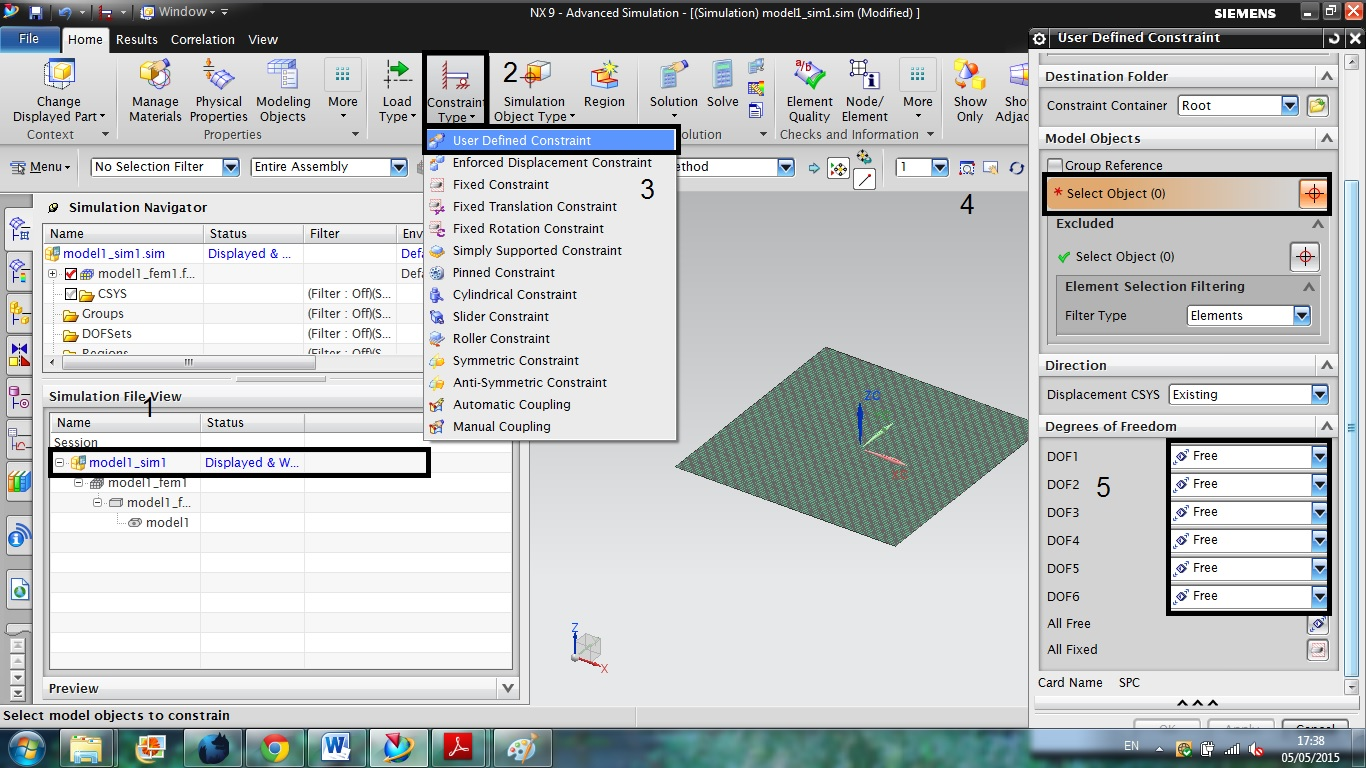10. ### Step 10:

DOF1: X / DOF 2 : Y/ DOF3: Z / DOF 4:Mx / DOF 5: My/ DOF 6: Mz

Chosen boundary condition:For simply supported boundary condition same as the R.Jones Book.

this part is significantly important, so if you want to do this tutorial please get enough information about the the kind of the boundary condition.

Point: The edge should not be fix in the applied forces direction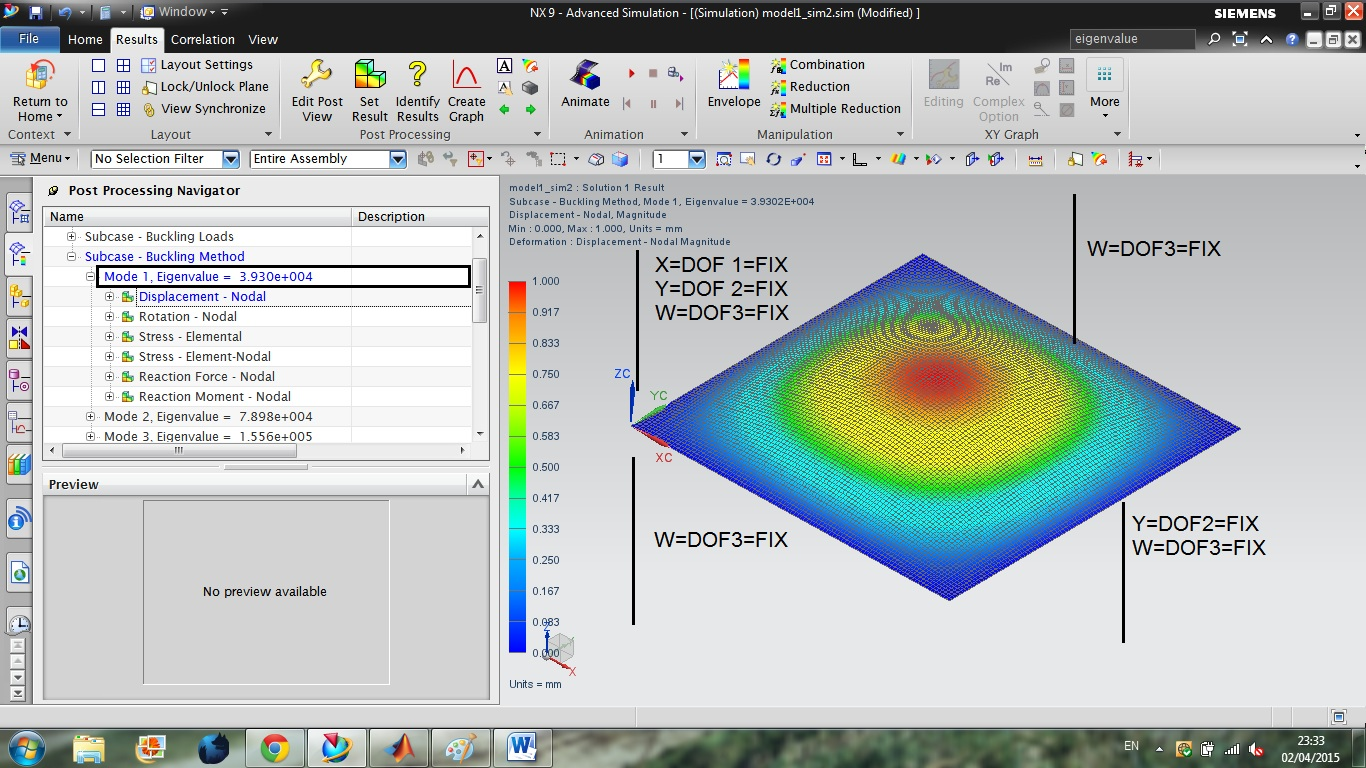11. ### Step 11:

I have just added this pic to show you how eigenvalue changes if you use different boundary condition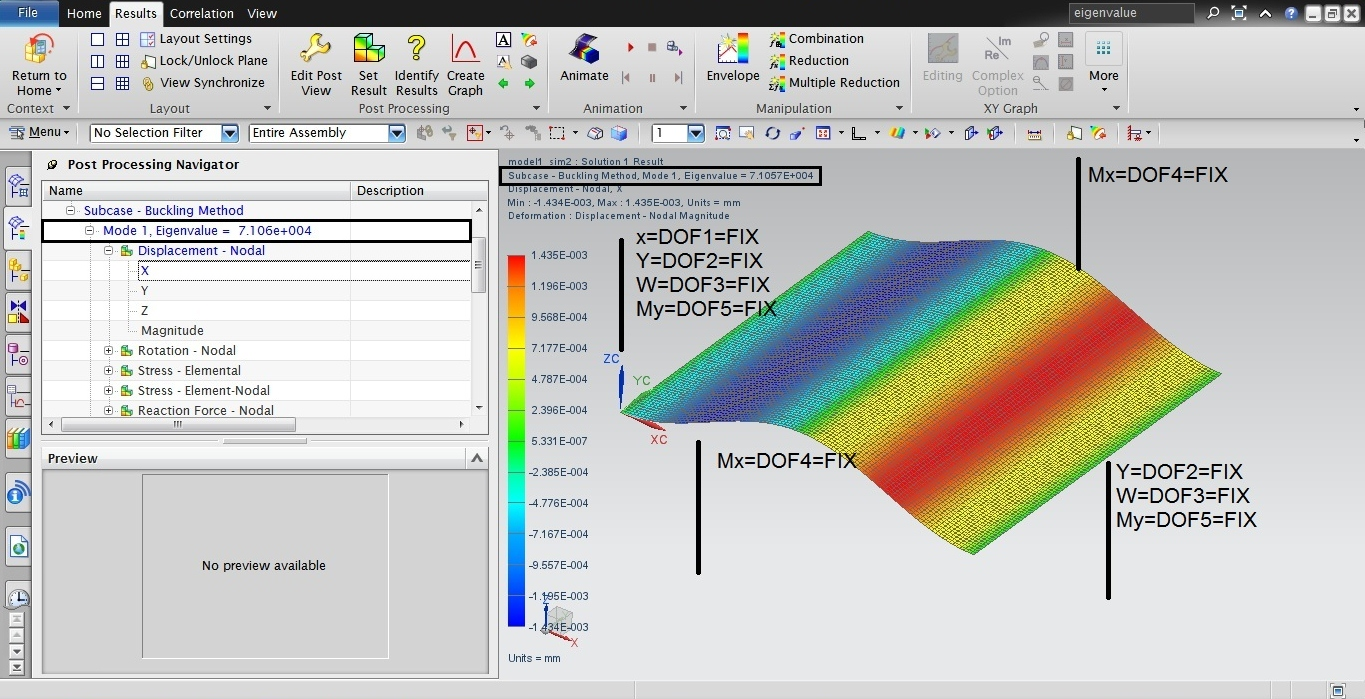12. ### Step 12:

you can choose the any amount for the applied forces, because after finding the eigenvalue this amount should be multiplied with the amount of forces. thereby, to simplify the question we consider 1 N for the applied forces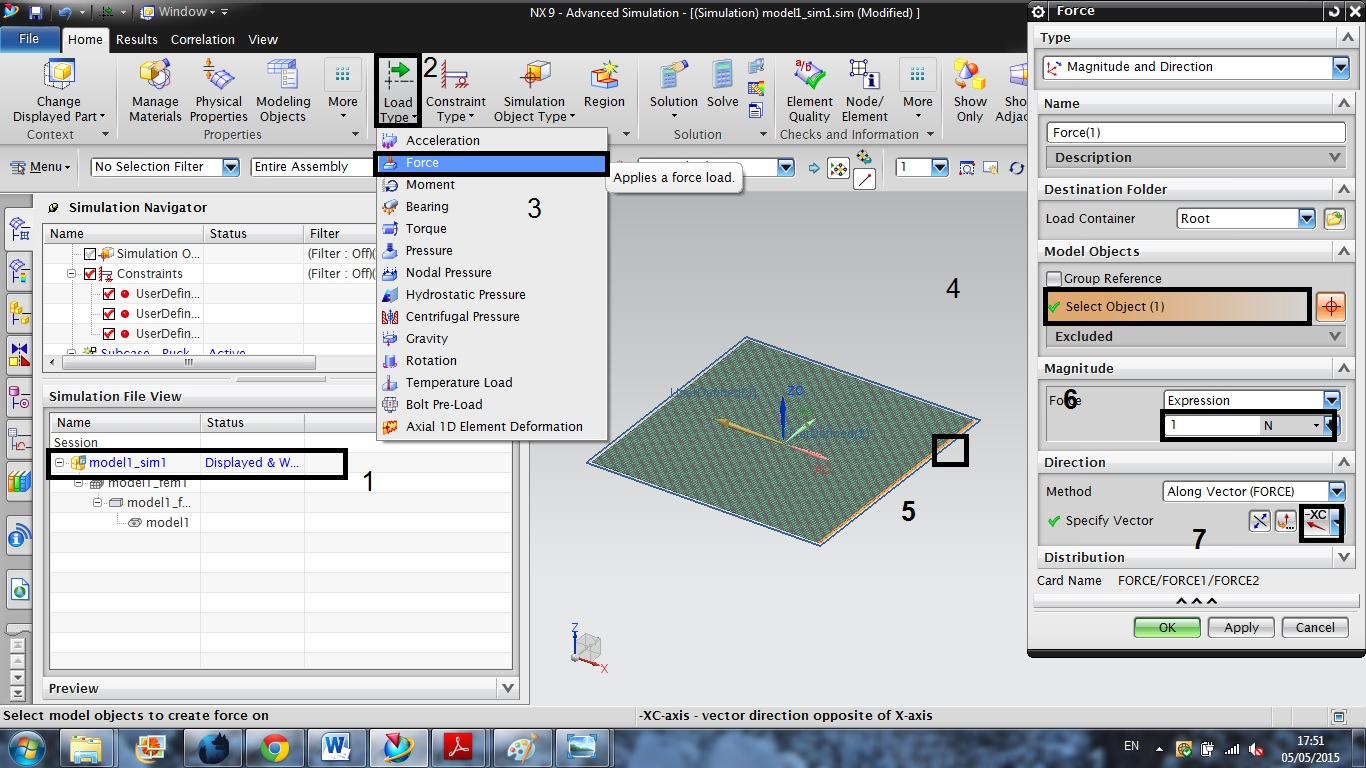13. ### Step 13:

CLICK ON SOLVE.

the first mode is critical buckling load. so we consider first eigenvalue as the critical buckling load